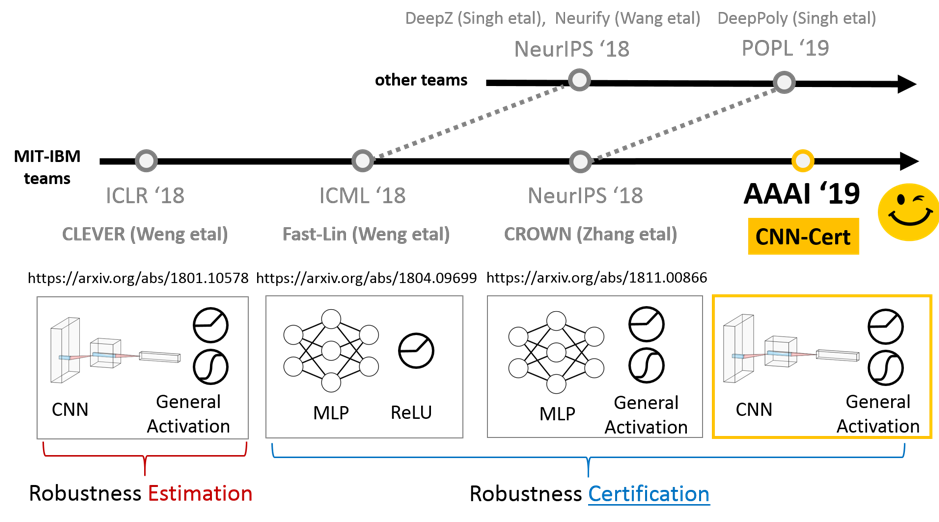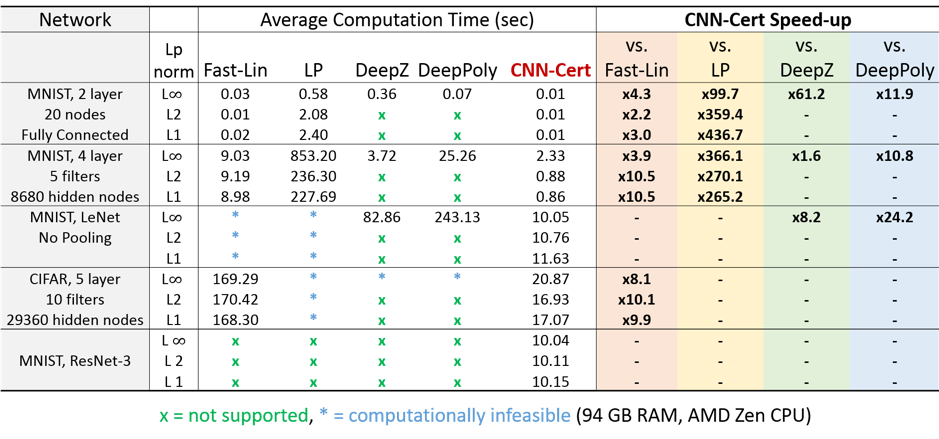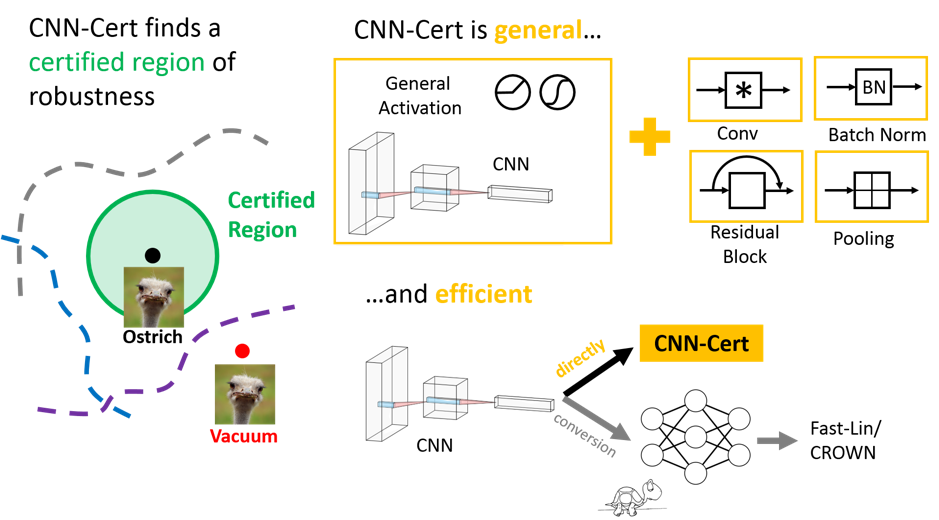# CNN-Cert: A Certified Measure of Robustness for Convolutional Neural NetworksFigure 1: An overview of robustness evaluation algorithms and robustness certification algorithms for neural networks.

#### Introduction

In this post, we briefly review a recent line of research on evaluating the robustness of neural network and its research progress, with a focus on the joint work accomplished by the MIT-IBM team. We start by giving an overview of this research stream. We then introduce three of our contributions along this line: the first robustness estimation score CLEVER, and two robustness lower bound certification algorithms, CROWN for neural networks with general activations and CNN-Cert for general convolutional neural network (CNN) architectures.

#### Overview

Assuming that attacks are Lp norm bounded, previous work has proven that finding the best possible certified robustness for ReLU networks is computationally intractable. However, several previous publications have attempted to find lower bounds on the robustness of neural networks and are summarized in Figure 1. Note that the concept of “robustness” here is defined as the minimum adversarial perturbation of a given test point and a given trained neural network classifier, which is a common setting in this research area. Researchers show that it is possible to find a lower bound on the robustness using norms of a network’s weight matrices, but these bounds are often very small for deep networks. Based on the publication date, Fast-Lin introduced non-trivial robustness bounds for fully connected networks (multilayer perceptrons). Mathematically, the later introduced DeepZ and Neurify methods provide equivalent or similar bounds to Fast-Lin. Next, the CROWN method extended the bounds to general activation functions and enhanced bounds on ReLU networks. Methodology-wise, for ReLU networks with only convolution layers CROWN and DeepPoly have an identical formulation. We also compare their numerical performances in Table 1.Table 1: Comparison between recent robustness certification algorithms. The improvement and speedup are calculated using results rounded to 4 decimal places. Due to space limits, we present numbers rounded to 2 decimal places. For LP formulations and its associated dual approach please refer to the works (Wong and Kolter) and (Dvijotham etal).

#### Our contribution 1: Evaluating robustness of neural network with CLEVER

At ICLR’18, we introduced a robustness metric called CLEVER (Cross Lipschitz Extreme Value for nEtwork Robustness) and its extension (CLEVER++) to help you evaluate how robust your trained neural network is to resist the Lp-norm based adversarial attacks. CLEVER has theoretical grounding based on Lipschitz continuity of the classifier model f and is scalable to state-of-the-art ImageNet neural network classifiers such as GoogleNet, ResNet and many others. However, due to the sampling-based approach of estimating Lipschitz constant with extreme value theory, the CLEVER score is an *estimate* of robustness but not a *certificate*.

#### Our contribution 2: Toward certifying robustness of neural networks with CROWN

The difference between CLEVER and a robustness certification algorithm is that a robustness certification algorithm will always deliver a certificate that guarantees to be smaller than the minimum adversarial distortion. This motivates MIT and IBM researchers to develop one of the very first neural network robustness certification algorithm — CROWN, which was just presented in NeurIPS 2018 in Montreal. CROWN is a general framework to certify neural networks based on linear and quadratic bounding techniques on the activation functions — CROWN is more flexible than its predecessor Fast-Lin and similar algorithms including DeepZ and Neurify in that CROWN features adaptive (non-parallel) activation bounds and can handle non-ReLU activations including but not limited to tanh, sigmoid, and arctan. It is worth noting that with the adaptive bounds feature, CROWN is able to tighten the robustness certificate provided by Fast-Lin by up to 20% on various MNIST and CIFAR fully-connected networks (MLPs). However, CROWN is limited to neural networks with fully-connected layers whereas in practice, convolutional neural networks with various architectures (e.g. pooling layers, residual blocks, batch-normalization layers) are more popular and prevalent.

Hence, this year at AAAI 2019, we propose a more general framework called CNN-Cert to help you quantify the level of robustness of your neural network classifiers with various building blocks including convolutional layers, residual blocks, pooling layers! This work will be published at AAAI 2019 and is selected for an oral presentation.Figure 2: An overview of CNN-Cert: CNN features robustness certification for general CNNs architectures and various building blocks and is more efficient than its predecessor Fast-Lin and CROWN algorithm.

#### Our contribution 3: Toward certifying robustness of general convolutional neural networks with CNN-Cert

CNN-Cert works on the same principle as its predecessors CROWN and Fast-Lin. The basic idea is to upper and lower bound the entire network with two linear functions of the input. This happens in an iterative process: bounds are first found for the first layer, and bounds for each successive layer are found using the bounds of the previous layers. These bounds are guaranteed to hold when the adversarial perturbation is bounded in Lp norm. What makes CNN-Cert different is that the bounds are represented in convolutional form. That is, the entire network is upper and lower bounded by two convolutional functions of the input. For a convolutional neural network, the complexity of this convolutional representation of the bounds is lower than a standard linear representation, and thus allows bounds to be computed more efficiently than previous approaches. These convolutional bounds can be found for networks with a variety of building blocks including but not limited to convolution layers, residual blocks, pooling and batch normalization. Indeed, any building block that can be bounded by convolutional functions can be incorporated into this framework. This means that CNN-Cert is both computationally efficient and general, as illustrated in Figure 2. The codes to reproduce CNN-Cert results can be found here.

#### Summary

Evaluating and quantifying the robustness of neural networks is certainly one of the most important research problem of deep neural networks as it can help us better understand the vulnerability of neural networks and also to form a basis of designing a more robust neural network in the future. In this article, we briefly reviewed three robustness evaluation and certification algorithms of our work and we refer interested readers to the following papers for more details:

#### Robustness scores

(1) CLEVER: https://arxiv.org/abs/1801.10578

(2) CLEVER++: https://arxiv.org/abs/1810.08640

#### Robustness certificates

(1) Fast-Lin:https://arxiv.org/abs/1804.09699

(2) CROWN: https://arxiv.org/abs/1811.00866

(3) CNN-Cert: https://arxiv.org/abs/1811.12395Authored by (L-R): Akhilan Boopathy (MIT), Lily Weng (MIT), Pin-Yu Chen (IBM Research), Sijia Liu (IBM Research), and Luca Daniel (MIT)